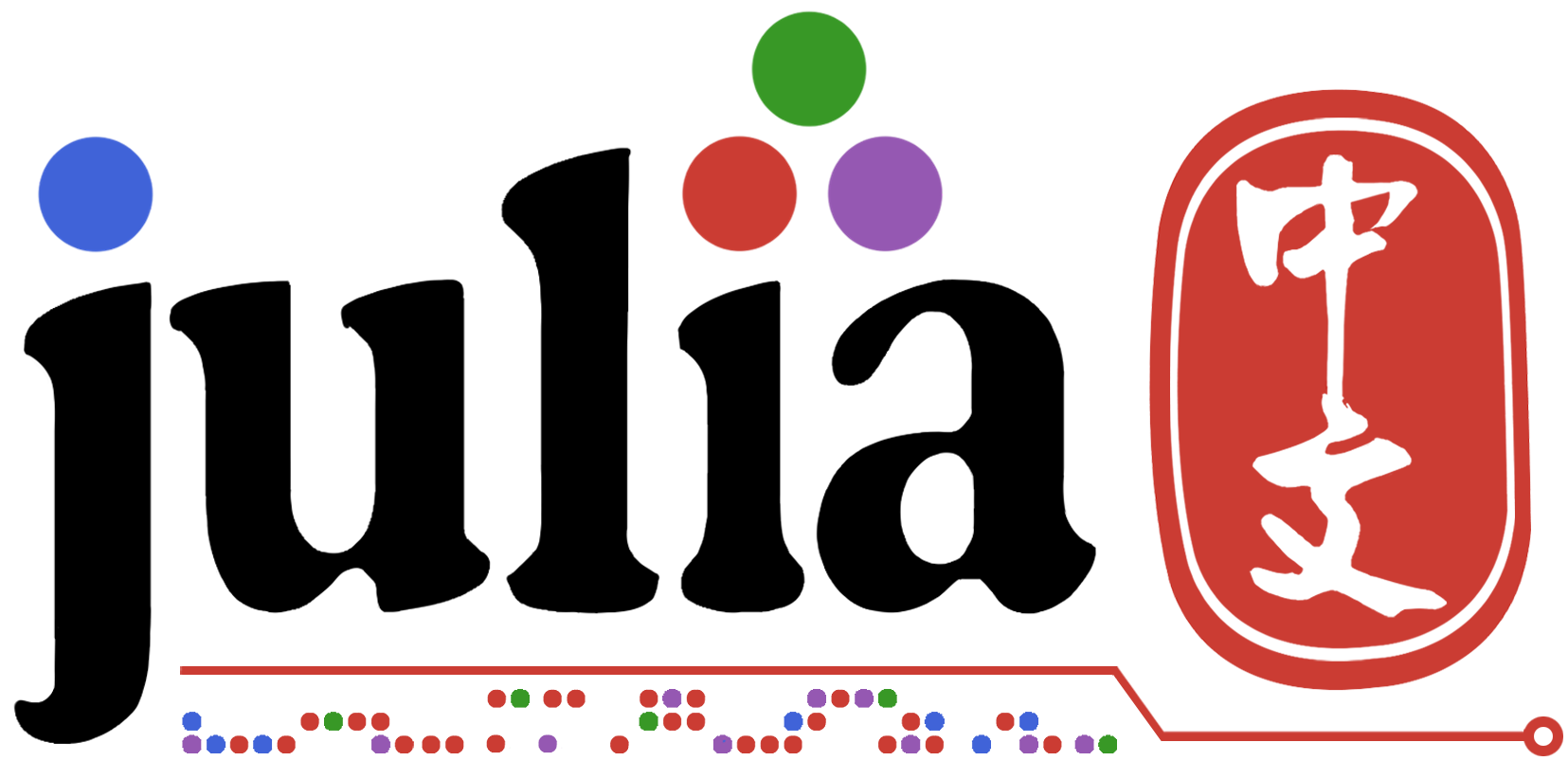# Fortran 与 Julia 之间的性能问题

Fortran经过这么多年的优化，而且是纯compiled language为什么Julia速度能超过它，是包的算法的优势还是语言本身的优势？

Julia用的LLVM也是多年优化的编译器。如果给编译器的信息一样，没有理由Julia会慢。Julia会推导出变量的数据类型再转化成LLVM IR（然后交给LLVM去优化并且产生native code）。

``````julia> function fib(n)
a, b = 1, 1
for i in 1:n-1
a, b = b, a+b
end
b
end
fib (generic function with 1 method)

julia> @code_typed fib(5)
CodeInfo(
3 1 ── %1  = (Base.sub_int)(n, 1)::Int64                                                                                              │╻     -
│    %2  = (Base.sle_int)(1, %1)::Bool                                                                                              ││╻╷╷╷  Type
│          (Base.sub_int)(%1, 1)::Int64                                                                                             │││╻     unitrange_last
│    %4  = (Base.ifelse)(%2, %1, 0)::Int64                                                                                          ││││
│    %5  = (Base.slt_int)(%4, 1)::Bool                                                                                              ││╻╷╷   isempty
└───       goto #3 if not %5                                                                                                        ││
2 ──       goto #4                                                                                                                  ││
3 ──       goto #4                                                                                                                  ││
4 ┄─ %9  = φ (#2 => true, #3 => false)::Bool                                                                                        │
│    %10 = φ (#3 => 1)::Int64                                                                                                       │
│    %11 = (Base.not_int)(%9)::Bool                                                                                                 │
└───       goto #10 if not %11                                                                                                      │
5 ┄─ %13 = φ (#4 => 1, #9 => %14)::Int64                                                                                            │
│    %14 = φ (#4 => 1, #9 => %16)::Int64                                                                                            │
│    %15 = φ (#4 => %10, #9 => %22)::Int64                                                                                          │
4 │    %16 = (Base.add_int)(%13, %14)::Int64                                                                                          │╻     +
│    %17 = (%15 === %4)::Bool                                                                                                       ││╻     ==
└───       goto #7 if not %17                                                                                                       ││
6 ──       goto #8                                                                                                                  ││
7 ── %20 = (Base.add_int)(%15, 1)::Int64                                                                                            ││╻     +
└───       goto #8                                                                                                                  │╻     iterate
8 ┄─ %22 = φ (#7 => %20)::Int64                                                                                                     │
│    %23 = φ (#6 => true, #7 => false)::Bool                                                                                        │
│    %24 = (Base.not_int)(%23)::Bool                                                                                                │
└───       goto #10 if not %24                                                                                                      │
9 ──       goto #5                                                                                                                  │
6 10 ─ %27 = φ (#8 => %16, #4 => 1)::Int64                                                                                            │
└───       return %27                                                                                                               │
) => Int64
``````

``````julia> using BenchmarkTools

julia> @btime fib(25)
1.882 ns (0 allocations: 0 bytes)
121393

julia> @code_native fib(25)
.text
testq   %rdi, %rdi
jle     L51
movl    \$1, %ecx
movl    \$1, %edx
nopw    %cs:(%rax,%rax)
movq    %rcx, %rax
movq    %rdx, %rcx
movq    %rax, %rdx
jne     L32
retq
L51:
movl    \$1, %eax
retq
nopl    (%rax)
;}
``````
3赞

1赞

5赞

C和Asm的BLAS库只能运算 `Float64`, `Float32`, `Complex{Float64}``Complex{Float32}` 。如果你用 https://github.com/JuliaPhysics/Measurements.jl 这种库就会很慢。

2赞

https://juejin.im/post/5b75911af265da27fc07a256

Julia的这个duck type比较彻底，没有别的给你用也。当然我觉得有时候会导致你发现某个类型虽然是 `AbstractMatrix` 但是有个什么方法没定义（因为可能作者本人不建议用这个矩阵类型做这种运算），但是不妨碍你用来干别的。

duck type目前我觉得使用体验还是非常好的。当然也不能说这个方案一定适合工程。

1赞

Julia未来的优化空间还有多大？

1赞

``````julia> using StaticArrays

julia> m2 = @SMatrix [ 1  3 ;
2  4. ];

julia> m1 = copy(m2);

julia> @code_native m2*m1;
.text
; Function * {
; Location: matrix_multiply.jl:9
; Function _mul; {
; Location: matrix_multiply.jl:75
; Function macro expansion; {
; Location: matrix_multiply.jl:78
; Function mul_unrolled; {
; Location: matrix_multiply.jl:123
; Function macro expansion; {
; Location: matrix_multiply.jl:138
; Function *; {
; Location: matrix_multiply.jl:9
vbroadcastf128  (%rsi), %ymm0   # ymm0 = mem[0,1,0,1]
vmovddup        (%rdx), %xmm1   # xmm1 = mem[0,0]
vmovddup        16(%rdx), %xmm2 # xmm2 = mem[0,0]
vinsertf128     \$1, %xmm2, %ymm1, %ymm1
vmulpd  %ymm1, %ymm0, %ymm0
vbroadcastf128  16(%rsi), %ymm1 # ymm1 = mem[0,1,0,1]
vmovddup        8(%rdx), %xmm2  # xmm2 = mem[0,0]
vmovddup        24(%rdx), %xmm3 # xmm3 = mem[0,0]
vinsertf128     \$1, %xmm3, %ymm2, %ymm2
vmulpd  %ymm2, %ymm1, %ymm1
;}}
; Function macro expansion; {
; Location: float.jl:395
;}}}}
vmovupd %ymm0, (%rdi)
movq    %rdi, %rax
vzeroupper
retq
nopw    %cs:(%rax,%rax)
;}
``````

``````julia> m2 = @SMatrix rand(8,8); m1 = copy(m2);

julia> @code_native m2*m1;
.text
; Function * {
; Location: matrix_multiply.jl:9
pushq   %r14
pushq   %rbx
subq    \$520, %rsp              # imm = 0x208
movq    %rdi, %rbx
; Function _mul; {
; Location: matrix_multiply.jl:75
; Function macro expansion; {
; Location: matrix_multiply.jl:78
movabsq \$julia_mul_unrolled_37105, %rax
leaq    8(%rsp), %r14
movq    %r14, %rdi
callq   *%rax
movabsq \$__memcpy_avx_unaligned_erms, %rax
;}}
movl    \$512, %edx              # imm = 0x200
movq    %rbx, %rdi
movq    %r14, %rsi
callq   *%rax
movq    %rbx, %rax
addq    \$520, %rsp              # imm = 0x208
popq    %rbx
popq    %r14
retq
nopw    %cs:(%rax,%rax)
;}
``````

``````julia> A = rand(50,50); q = qr(A);

julia> q.T |> size
(36, 50)
``````

``````julia> m2 = @SMatrix rand(36,36); m1 = copy(m2);

julia> m3 = rand(36,36); m4=copy(m3);

julia> @btime \$m3*\$m4;
6.155 μs (1 allocation: 10.25 KiB)

julia> @btime \$m2*\$m1;
7.136 μs (0 allocations: 0 bytes)
``````
1赞

Julia的SIMD没有OpenBLAS或者BLIS手写的好。他也是从矩阵大小上下工夫的。他的库算出比较好的block大小，然后生成的kernel。相比之下BLIS或者OpenBLAS只有4种microkernel。

okay，也就是说他可以生成大量的kernel？所以在他展示的benchmark里面更好？大矩阵应该就没有什么差别了吧？

BLAS在CPU上没有什么优化的空间了。基本上都能在大矩阵上得到几乎CPU的峰值速度的。Julia可以优化的方向就是更加紧密地连接底层Level 3 BLAS和高层线性代数（LAPACK）的联系。毕竟只要调整高层线性代数的block大小，并且让BLAS对那个大小优化，那么可以让Julia的高层线性代数非常的快。

1赞

Okay, 不过感觉我们现在的体验还是在利用type优化一些特别的矩阵类型上，也比较好实现。然而其实我一直没弄明白为啥 `StaticArray` 没我们手写的permutation好。我改天再开个帖子讨论好了。

StaticArrays are built on tuples which have inference limits. As the compiler improves this could improve. One big thing that is missing is the ability to recognize tuples as homogeneous so that full inference doesn’t have to happen.

3赞